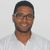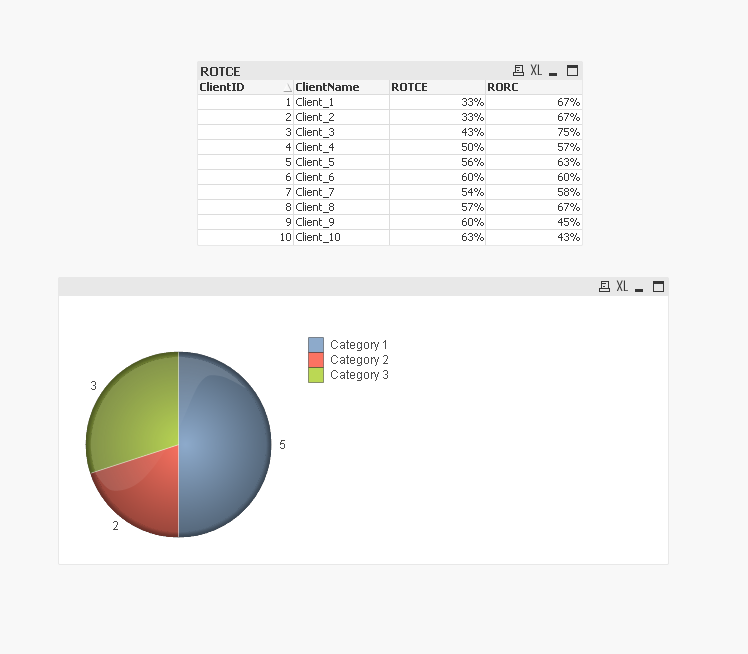# QlikView App Dev

Discussion Board for collaboration related to QlikView App Development.

Announcements
QlikWorld 2023, a live, in-person thrill ride. Save \$300 before February 6: REGISTER NOW!
cancel
Showing results for
Did you mean:Contributor

## Need help with Expression

We calculate 2 metrics
1) ROTCE = Net_Income/Total_TCE
2) RORC = Net_Income/Total_RC

We need to Count all the clients in 4 categories

• Category 1 - ROTCE >= 50% AND RORC >= 50%
• Category 2 - ROTCE >= 50% AND RORC < 50%
• Category 3 - ROTCE < 50% AND RORC >= 50%
• Category 4 - ROTCE < 50% AND RORC < 50%

and create a Pie Chart or Mecco chart showing Total Clients count by Category.

Please guide us on how to create an expression.

1 Solution

Accepted SolutionsMVP

attached Qlikview one solution :

ROTCE

``sum({<MetricDesc={'Net_Income'}>} Value)/sum({<MetricDesc={'Total_TCE'}>} Value)``

RORC

``sum({<MetricDesc={'Net_Income'}>} Value)/sum({<MetricDesc={'Total_RC'}>} Value)``

and the pie chart

output :Regards,
Taoufiq ZARRA

"Please LIKE posts and "Accept as Solution" if the provided solution is helpful "

(you can mark up to 3 "solutions") 😉MVP

attached Qlikview one solution :

ROTCE

``sum({<MetricDesc={'Net_Income'}>} Value)/sum({<MetricDesc={'Total_TCE'}>} Value)``

RORC

``sum({<MetricDesc={'Net_Income'}>} Value)/sum({<MetricDesc={'Total_RC'}>} Value)``

and the pie chart

output :Regards,
Taoufiq ZARRA

"Please LIKE posts and "Accept as Solution" if the provided solution is helpful "

(you can mark up to 3 "solutions") 😉Community Browser